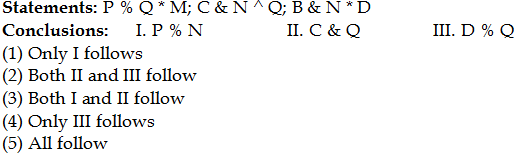## PREPARATORY QUESTIONS

### EQUAL-INEQUAL

#### PREPARATORY SET-03 (HARD)

Direction (Qs.1 to 5): In the following questions, the symbols %, \$, ^, * and & are used with the following meanings as illustrated below, answer the questions accordingly.

‘A % B’ means ‘A is greater than B’

‘A % B’ means ‘A is greater than B’

‘A * B’ means ‘A is neither greater than nor equal to B’

‘A & B’ means ‘A is either greater than or equal to B’

‘A ^ B’ means ‘A is neither smaller than nor greater than B’

Now in each of the following questions assuming the given statement to be true, find which of the conclusions given below them is/are definitely true and give your answer accordingly.

Question No : 1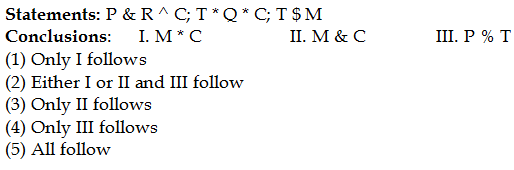Question No : 2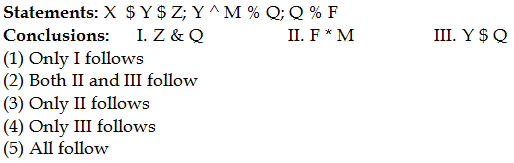Question No : 3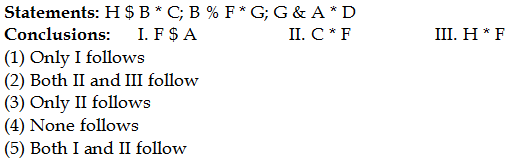Question No : 4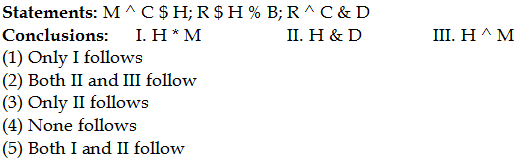Question No : 5